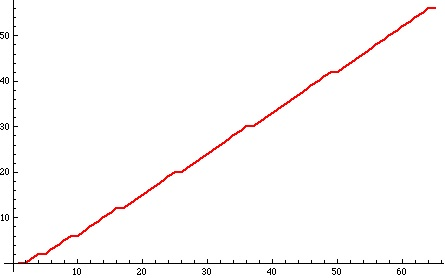# When is an integer a square?

Posted by: Gary Ernest Davis on: November 30, 2010

This post came out of a conversation with James Tanton (@jamestanton) about squares of integers being exactly the integers not represented by rounding$n+\sqrt{n}$ for$n$ an integer (see here for background).

To say that rounding$n+\sqrt{n}$ yields an integer$k$ is to say that:$k-\frac{1}{2} < n+\sqrt{n} < k+\frac{1}{2}$……………………………. (*)

Subtracting$n$ from the inequalities in (*) and squaring – as we can do and preserve the inequalities because the resulting left hand expression is positive – we get two quadratic inequalities for$n$.

After some consideration of these inequalities I was lead to consider the function$F(k):=\textrm{Floor}[k-\sqrt{k-1/4}]$.

Below is a plot of this function for$1 \leq k \leq 65$:What is striking is that there are little steps in the graph.

What’s more striking is that these steps occur precisely when$k$ is a square.

In other words this graph, and much more numerical evidence, suggest that an integer$k$ is a square exactly when$F(k)=F(k+1)$.

I believe this, from the overwhelming numerical evidence, but I cannot yet see why.

Maybe someone else can?

#### Postscript

Nick Hobson (@quintic) provided a nice argument, in the comments below,Â  that shows$k$ is a square exactly when$F(k)=F(k+1)$.

### 3 Responses to "When is an integer a square?"I don’t have an answer, but I noted this:

(k – 1/4k)^.5 is either a whole number or a whole number + .5 when k is 3 12 27 48 75 ….

where the second differences are all 6.

Not sure what (if anything) this adds to the solutionFloor(k – âˆš(k – Â¼)) = Floor(k) – Floor(âˆš(k – Â¼)) – 1 = k – 1 – Floor(âˆš(k – Â¼)), for all k > 0, since âˆš(k – Â¼) is never an integer (because k – Â¼ is never the square of an integer.)

So F(k+1) – F(k) = 1 – (Floor(âˆš(k + Â¾)) – Floor(âˆš(k – Â¼))), which is clearly equal to 1 unless k is a perfect square, when it equals 0.Thanks Nick. That’s great.